# How to Teach Fraction Division….Another Way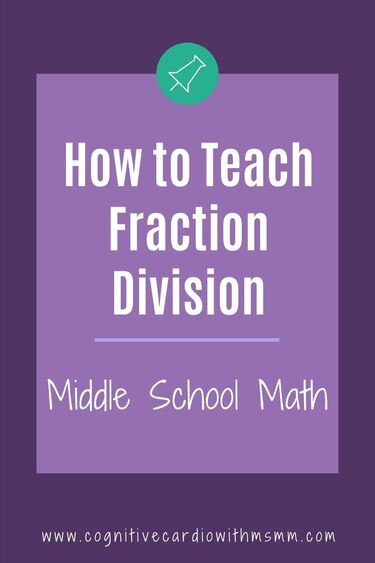## Fraction Division in Middle School Math

Have you ever taught fraction division to the students in your middle school math classes, only to find them “flipping” the wrong number?

Students may have been taught to “skip, flip, flip,” “invert and multiply,” or “multiply by the reciprocal.”

They may have “learned” the steps or learned a fun fraction division song to help them remember; but somehow they still flip the wrong one or they forget to flip at all.

​OR students change a mixed number into an improper fraction and seem to subconsciously think that since they did something to that mixed number, the flipping had already occurred…and then they don’t flip anything.

Why does this happen? I’m going to say that it happens because students don’t understand why they’re flipping anything – it doesn’t mean anything to them.

## Fraction Division Using Common Denominators

So, I started using another way to teach fraction division – perhaps you’ve heard of it, or you already use it.
I never learned it this way as a student, but I like it, and it makes more sense to some students. I learned this method when I had a student teacher a few years back. She was teaching the fraction unit, and when her supervisor came in to observe and discuss, she asked if I had ever taught fraction division using common denominators. Having only learned (and then taught) to multiply by the reciprocal, of course I said no.

The next time she visited, she brought me a page from a textbook that explained dividing fractions using common denominators. These are the steps:

• Step 1: Find common denominators, just as when adding and subtracting and then make equivalent fractions (students are already used to doing this – hopefully).
• Step 2: Create a new fraction with the numerator of the first fraction over the numerator of the second fraction…this is your answer.
• Done (unless you need to simplify)!

​I was shocked – it seemed SO simple!

## Fraction Division Example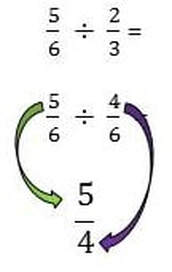Check out this example – it’s a simple one, for starters:
5/6 divided by 2/3.

1. ​Find the common denominator of 6 and 3, which is 6. This gives you 5/6 divided by 4/6.
2. The first numerator (5) becomes the numerator in the answer. The second numerator (4) becomes the denominator.
3. Simplify

## Fraction Division Example 2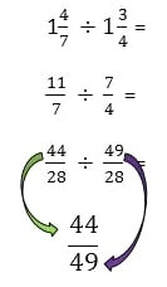Let’s look at another one, with mixed numbers:
1_4/7 divided by 1_3/4.

1. Convert the mixed numbers to improper fractions, which gives you 11/7 divided by 7/4.
2. Find the common denominator of 28 and make equivalent fractions. This gives you 44/28 divided by 49/28.
3. The first numerator (44) becomes the numerator in the answer. The second numerator (49) becomes the denominator.
4. No simplifying, in this case.

## Why Does Using Common Denominators Work?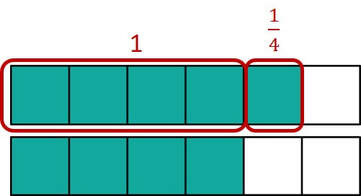Once the denominators are the same, we’re dealing with fraction pieces that are the same size.

When we look at 5/6 ÷ 4/6, there are 5 pieces and 4 pieces that are the same size (our numerators).
So, we’re really looking at 5
÷ 4, or how many times 4 fits into 5.

## Student Response to Dividing Fractions with Common Denominators

I teach both methods to my sixth-graders. Some really like it…it makes sense to them.
Others stick to the flipping method, but I don’t know if this is because they like it better or because it was the first way they learned it…..most of them had been taught something about fraction division in 5th grade.

## Fraction Division Using the Reciprocal

Multiplying by the reciprocal – if students are going to use it, I think it’s important that they understand WHY it works.
It may be tough for them to understand in 5th or 6th grade, but if they learn the common denominator method first, the proof may then make more sense to them.
I found a great article on the NCTM website that uses the common denominator method to prove why multiplying by the reciprocal works – check it out, if you have time:-)

## ​Fraction Division Math Wheels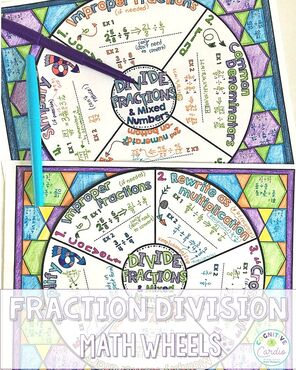Not too long ago, I made two math wheels, to use to teach both methods of dividing fractions – taking notes with the wheel will be more fun!
Students can save their wheel to use as a reference throughout the year.

​What do you think? Do you see any advantages or disadvantages to teaching fraction division using common denominators?

## Resources to teach and practice fraction division

Interested in more fraction and fraction operation content?
Check out the course,
 Grab this free fraction operations math wheel (plus other fraction goodies) when you join the email community!

## EllieWelcome to Cognitive Cardio Math! I’m Ellie, a wife, mom, grandma, and dog ‘mom,’ and I’ve spent just about my whole life in school! With nearly 30 years in education, I’ve taught:

• All subject areas in 4th and 5th grades
• Math, ELA, and science in 6th grade (middle school)

I’ve been creating resources for teachers since 2012 and have worked in the elearning industry for about five years as well!